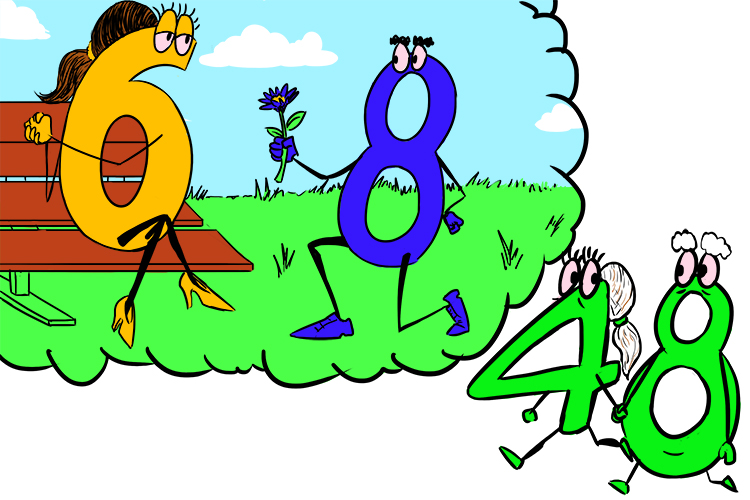# 6 Times table

From 1times2 right up to 12times12, the only multiplication that actually has to be learnt is:

6xx8=48

See mnemonic at the bottom.

And remember that multiplication works both ways.

2times6 = see 2 times table

3times6 = see 3 times table

4times6 = see 4 times table

5times6 = see 5 times table

6times6 = see multiply by itself mnemonic = 36

7times6 = 6times7 which is 6times6=36  then  36+6=42

8times6 = see mnemonic at the bottom

9times6 = see 9 times table

10times6 = see 10 times table

11times6 = see 11 times table

12times6 = see 12 times table

## Help to remember 8 x 6 = 48

Use this rhyme to help you remember 6 xx 8 = 48

Six and eight went on a date and didn't come back until they were 48.6 and 8 = 48